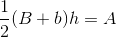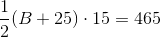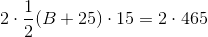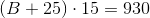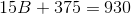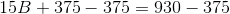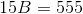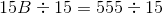# ISEE Upper Level Math : How to find the length of the side of a trapezoid

## Example Questions

### Example Question #9 : Trapezoids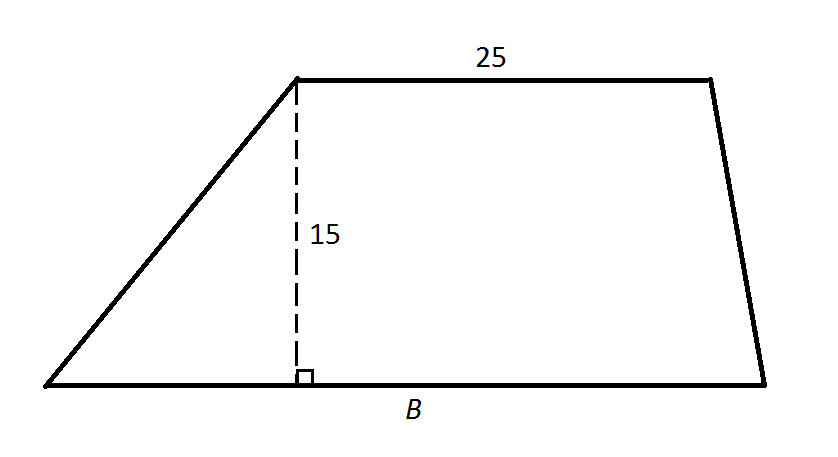Note: Figure NOT drawn to scale.

The area of the above trapezoid is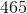. What is?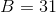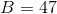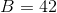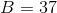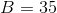Explanation:

Substitute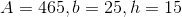into the formula for the area of a trapezoid: